# Translativity of a summation method

(diff) ← Older revision | Latest revision (diff) | Newer revision → (diff)

The property of the method consisting in the preservation of summability of a series after adding to or deleting from it a finite number of terms. More precisely, a summation methodis said to be translative if the summability of the series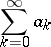to the sumimplies that the series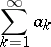is summable by the same method to the sum, and conversely. For a summation methoddefined by transformation of the sequenceinto a sequence or function, the property of translativity consists of the equivalence of the conditions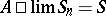andIf the summation method is defined by a regular matrix(cf. Regular summation methods), then this means that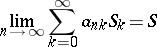(1)

always implies that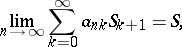(2)

and conversely. In cases when such an inference only holds in one direction, the method is called right translative if (1) implies (2) but the converse is false, or left translative if (2) implies (1) but the converse is false.

Many widely used summation methods have the property of translativity; for example, the Cesàro summation methodsfor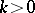, the Riesz summation methodforand the Abel summation method are translative; the Borel summation method is left translative.

How to Cite This Entry:
Translativity of a summation method. Encyclopedia of Mathematics. URL: http://encyclopediaofmath.org/index.php?title=Translativity_of_a_summation_method&oldid=37081
This article was adapted from an original article by I.I. Volkov (originator), which appeared in Encyclopedia of Mathematics - ISBN 1402006098. See original article